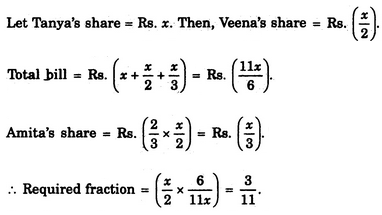# Verbal Reasoning - Arithmetic Reasoning

### Exercise :: Arithmetic Reasoning - Section 1

36.

Three friends had dinner at a restaurant. When the bill was received, Amita paid 2/3 as much as Veena paid and Veena paid 1/2 as much as Tanya paid. What faction of the bill did Veena pay ?

 A. 1/3 B. 3/11 C. 12/13 D. 5/8

Explanation:37.

In a class, 20% of the members own only two cars each, 40% of the remaining own three cars each and the remaining members own only one car each. Which of the following statements is definitely true from the given statements ?

 A. Only 20% of the total members own three cars each. B. 48% of the total members own only one car each. C. 60% of the total members own at least two cars each. D. 80% of the total members own at least one car. E. None of these

Explanation:

Let total number of members be 100,

Then, number of members owning only 2 cars = 20.

Number of members owning 3 cars = 40% of 80 = 32.

Number of members owning only 1 car = 100 - (20 + 32) = 48.

Thus, 48% of the total members own one car each.

38.

When Rahul was born, his father was 32 years older than his brother and his mother was 25 years older than his sister. If Rahul's brother is 6 years older than him and his mother is 3 years younger than his father, how old was Rahul's sister when he was born ?

 A. 7 years B. 10 years C. 14 years D. 19 years

Explanation:

When Rahul was born, his brother's age = 6 years; his father's age = (6 + 32) years = 38 years, his mother's age = (38 - 3) years = 35 years; his sister's age = (35 - 25) years = 10 years.

39.

A certain number of horses and an equal number of men are going somewhere. Half of the owners are on their horses' back while the remaining ones are walking along leading their horses. If the number of legs walking on the ground is 70, how many horses are there ?

 A. 10 B. 12 C. 14 D. 16

Explanation:

Let number of horses = number of men = x.

Then, number of legs = 4x + 2 x (x/2) = 5x.

So, 5X = 70 or x = 14.

40.

Ravi's brother is 3 years senior to him. His father was 28 years of age when his sister was born while his mother was 26 years of age when he was born. If his sister was 4 years of age when his brother was born, what were the ages of Ravi's father and mother respectively when his brother was born ?

 A. 32 years, 23 years B. 32 years, 29 years C. 35 years, 29 years D. 35 years, 33 years

Explanation:

When Ravi's brother was born, let Ravi's father's age = x years and mother's age = y years.

Then, sister's age = (x - 28) years. So, x - 28 = 4 or x = 32.

Ravi's age = (y - 26) years. Age of Ravi's brother = (y - 26 + 3) years = (y - 23) years.

Now, when Ravi's brother was born, his age = 0 i.e. y - 23 = 0 or y = 23.